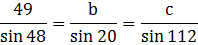### Sample ProblemUse the Law of Sines to solve this triangle. Round the lengths of the sides to three decimal places.

A= °

Side b= ft

Side c= ft

#### Solution

A=180°-20°-112°=48°49/sin 48 * (sin 20)=b=22.551 ft

49/sin 48 * (sin 112)=c=61.135 ft